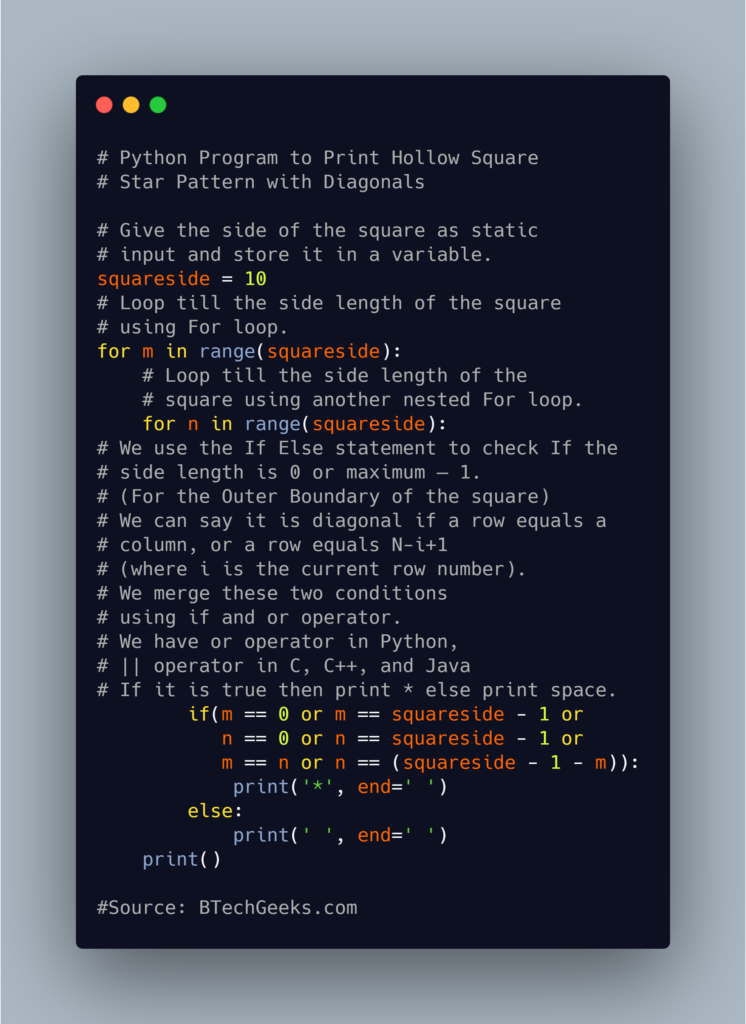# Python Program to Print Hollow Square Star With Diagonals

Beginners and experienced programmers can rely on these Best Java Programs Examples and code various basic and complex logics in the Java programming language with ease.

Given the sides of the square, the task is to print the hollow Square star pattern with diagonals in C, C++, and Python.

Examples:

Example1:

Input:

given number of sides of square =10

Output:

* * * * * * * * * *
* *                * *
*   *            *   *
*     *        *     *
*       *   *        *
*       *   *        *
*     *       *      *
*   *           *    *
* *                * *
* * * * * * * * * *

Example2:

Input:

given number of sides of square =10
given character to print =$ Output: $                                                                                                       $ ## Program to Print Hollow Square Star Pattern with Diagonals in C, C++, and Python Below are the ways to print the hollow square star with Diagonals pattern in C, C++, and Python. ### Method #1: Using For loop (Star Character) Approach: • Give the side of the square as static input and store it in a variable. • Loop till the side length of the square using For loop. • Loop till the side length of the square using another nested For loop. • We use the If Else statement to check If the side length is 0 or maximum – 1. (For the Outer Boundary of the square) • We can say it is diagonal if a row equals a column, or a row equals N-i+1 (where i is the current row number). • We merge these two conditions using if and or operator. • We have or operator in Python,|| operator in C, C++, and Java • If it is true then print * else print space. • The Exit of the Program. 1) Python Implementation Below is the implementation: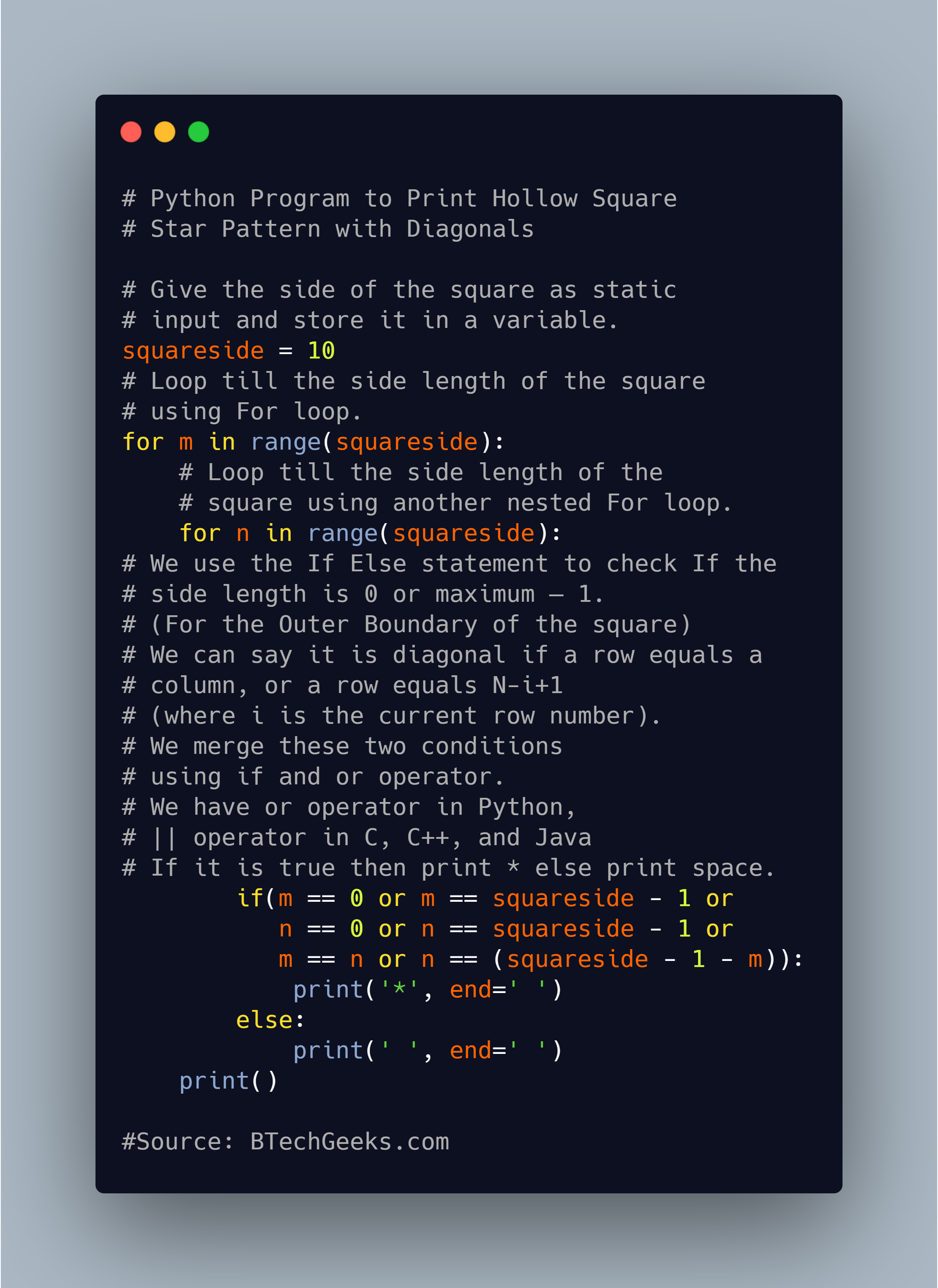# Give the side of the square as static input and store it in a variable. squareside = 10 # Loop till the side length of the square using For loop. for m in range(squareside): # Loop till the side length of the square using another nested For loop. for n in range(squareside): # We use the If Else statement to check If the side length is 0 or maximum – 1. (For the Outer Boundary of the square) # We can say it is diagonal if a row equals a column, or a row equals N-i+1 (where i is the current row number). # We merge these two conditions using if and or operator. # We have or operator in Python,|| operator in C, C++, and Java # If it is true then print * else print space. if(m == 0 or m == squareside - 1 or n == 0 or n == squareside - 1 or m == n or n == (squareside - 1 - m)): print('*', end=' ') else: print(' ', end=' ') print()  Output: * * * * * * * * * * * * * * * * * * * * * * * * * * * * * * * * * * * * * * * * * * * * * * * * * * * * 2) C++Implementation Below is the implementation: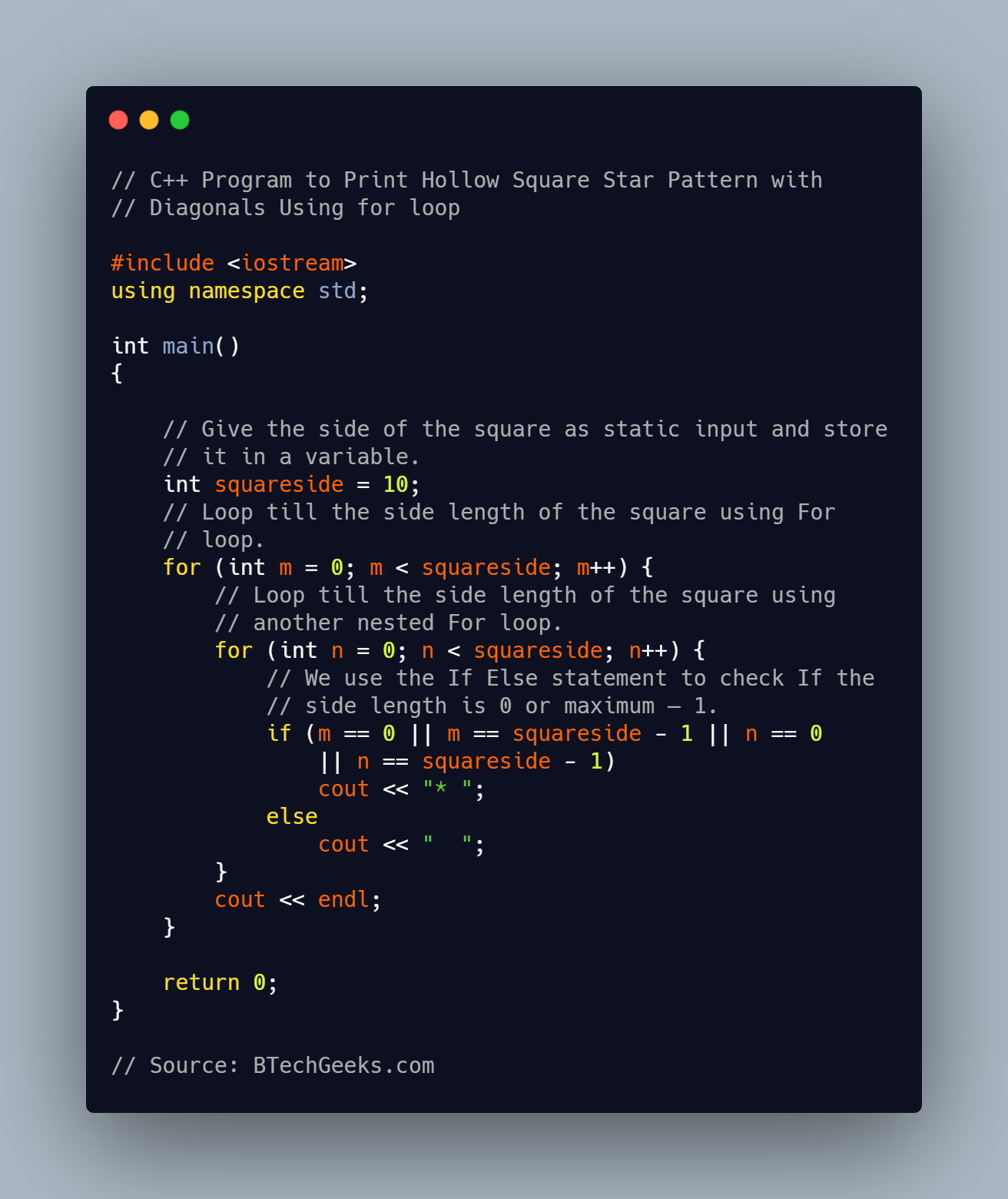#include <iostream> using namespace std; int main() { // Give the side of the square as static input and store // it in a variable. int squareside = 10; // Loop till the side length of the square using For // loop. for (int m = 0; m < squareside; m++) { // Loop till the side length of the square using // another nested For loop. for (int n = 0; n < squareside; n++) { // We use the If Else statement to check If the // side length is 0 or maximum – 1. if (m == 0 || m == squareside - 1 || n == 0 || n == squareside - 1) cout << "* "; else cout << " "; } cout << endl; } return 0; } Output: * * * * * * * * * * * * * * * * * * * * * * * * * * * * * * * * * * * * * * * * * * * * * * * * * * * * 3) C Implementation Below is the implementation: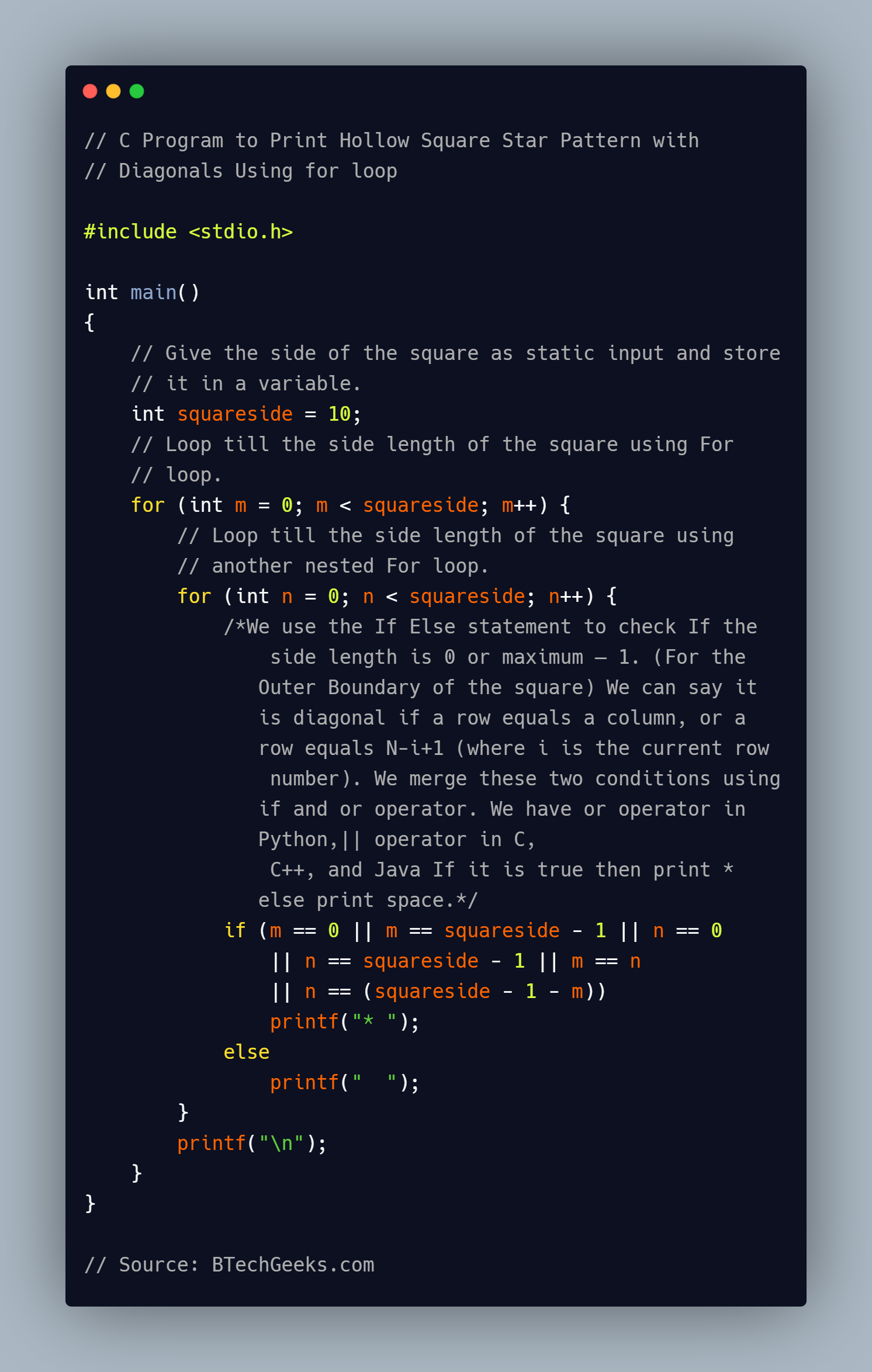#include <stdio.h> int main() { // Give the side of the square as static input and store // it in a variable. int squareside = 10; // Loop till the side length of the square using For // loop. for (int m = 0; m < squareside; m++) { // Loop till the side length of the square using // another nested For loop. for (int n = 0; n < squareside; n++) { /*We use the If Else statement to check If the side length is 0 or maximum – 1. (For the Outer Boundary of the square) We can say it is diagonal if a row equals a column, or a row equals N-i+1 (where i is the current row number). We merge these two conditions using if and or operator. We have or operator in Python,|| operator in C, C++, and Java If it is true then print * else print space.*/ if (m == 0 || m == squareside - 1 || n == 0 || n == squareside - 1 || m == n || n == (squareside - 1 - m)) printf("* "); else printf(" "); } printf("\n"); } } Output: * * * * * * * * * * * * * * * * * * * * * * * * * * * * * * * * * * * * * * * * * * * * * * * * * * * * ### Method #2: Using For Loop (User Character) Approach: • Give the side of the square as user input and store it in a variable. • Scan the character to print as user input and store it in a variable. • Loop till the side length of the square using For loop. • Loop till the side length of the square using another nested For loop. • We use the If Else statement to check If the side length is 0 or maximum – 1. • We can say it is diagonal if a row equals a column, or a row equals N-i+1 (where i is the current row number). • We merge these two conditions using if and or operator. • We have or operator in Python,|| operator in C, C++, and Java • If it is true then print given character else print space. • The Exit of the Program. 1) Python Implementation • Give the number of sides of the square as user input using int(input()) and store it in a variable. • Give the Character as user input using input() and store it in another variable. Below is the implementation: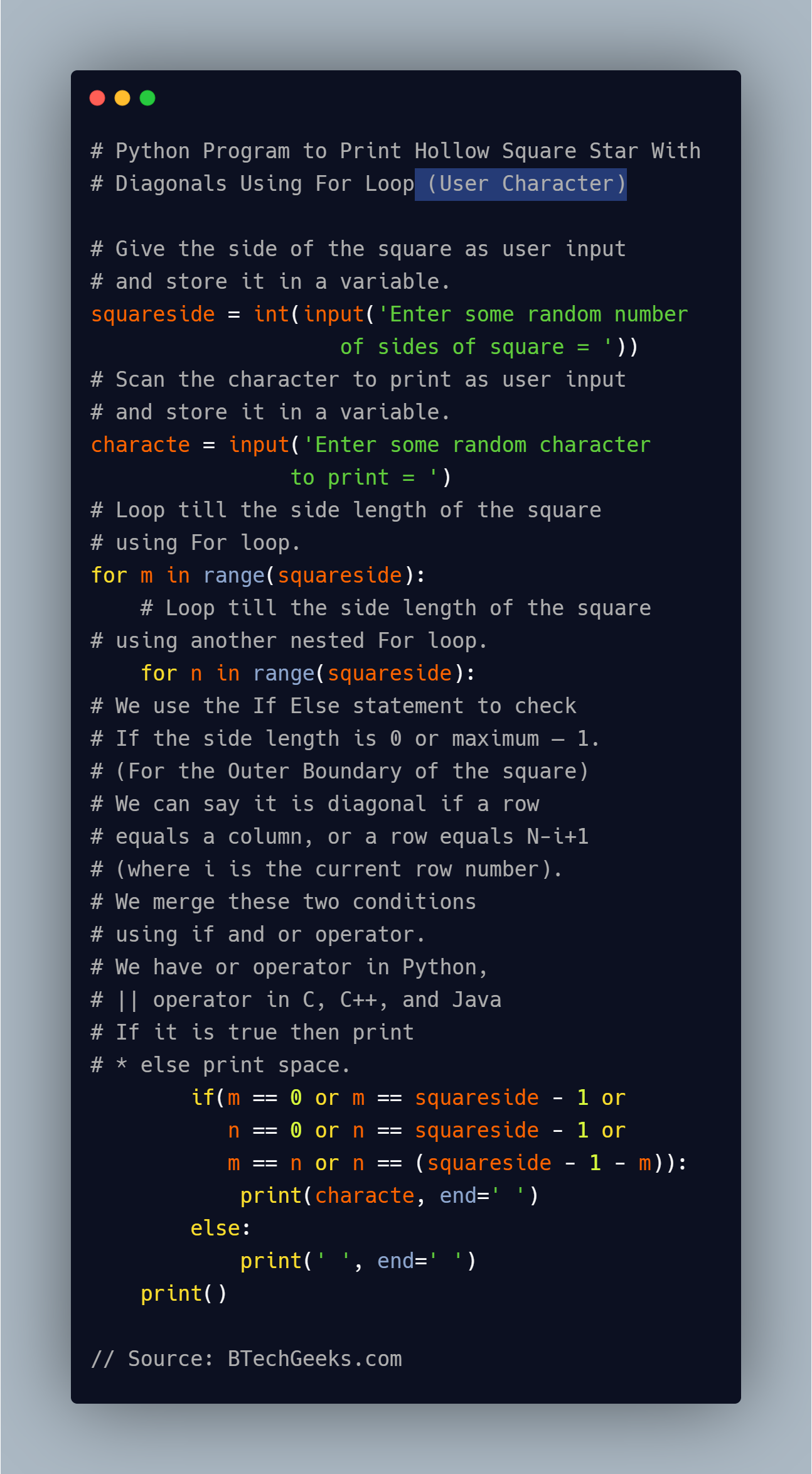# Give the side of the square as user input and store it in a variable. squareside = int(input('Enter some random number of sides of square = ')) # Scan the character to print as user input and store it in a variable. characte = input('Enter some random character to print = ') # Loop till the side length of the square using For loop. for m in range(squareside): # Loop till the side length of the square using another nested For loop. for n in range(squareside): # We use the If Else statement to check If the side length is 0 or maximum – 1. (For the Outer Boundary of the square) # We can say it is diagonal if a row equals a column, or a row equals N-i+1 (where i is the current row number). # We merge these two conditions using if and or operator. # We have or operator in Python,|| operator in C, C++, and Java # If it is true then print * else print space. if(m == 0 or m == squareside - 1 or n == 0 or n == squareside - 1 or m == n or n == (squareside - 1 - m)): print(characte, end=' ') else: print(' ', end=' ') print()  Output: Enter some random number of sides of square = 10 Enter some random character to print =$
    
                   
               
        
   
 
   
          
                
    

2) C++Implementation

• Give the number of sides of the square as user input using cin and store it in a variable.
• Create a character variable.
• Give the character as user input using cin and store it in another variable.

Below is the implementation: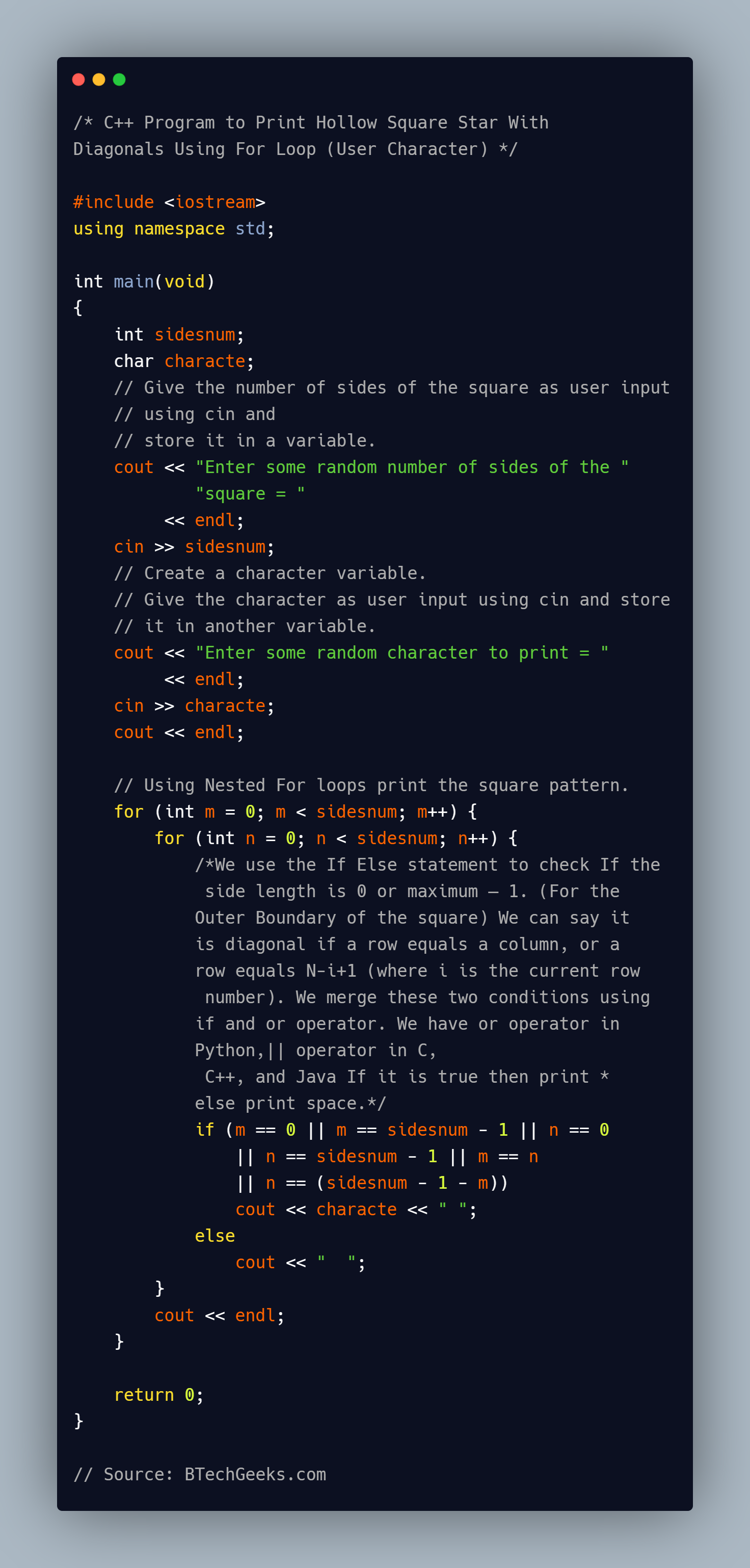#include <iostream>
using namespace std;

int main(void)
{
int sidesnum;
char characte;
// Give the number of sides of the square as user input
// using cin and
// store it in a variable.
cout << "Enter some random number of sides of the "
"square = "
<< endl;
cin >> sidesnum;
// Create a character variable.
// Give the character as user input using cin and store
// it in another variable.
cout << "Enter some random character to print = "
<< endl;
cin >> characte;
cout << endl;

// Using Nested For loops print the square pattern.
for (int m = 0; m < sidesnum; m++) {
for (int n = 0; n < sidesnum; n++) {
/*We use the If Else statement to check If the
side length is 0 or maximum – 1. (For the
Outer Boundary of the square) We can say it
is diagonal if a row equals a column, or a
row equals N-i+1 (where i is the current row
number). We merge these two conditions using
if and or operator. We have or operator in
Python,|| operator in C,
C++, and Java If it is true then print *
else print space.*/
if (m == 0 || m == sidesnum - 1 || n == 0
|| n == sidesnum - 1 || m == n
|| n == (sidesnum - 1 - m))
cout << characte << " ";
else
cout << "  ";
}
cout << endl;
}

return 0;
}

Output:

Enter some random number of sides of the square =
10
Enter some random character to print =
                                                                                                       $ 3) C Implementation • Give the number of sides of the square as user input using scanf and store it in a variable. • Create a character variable. • Give the character as user input using scanf and store it in another variable. Below is the implementation: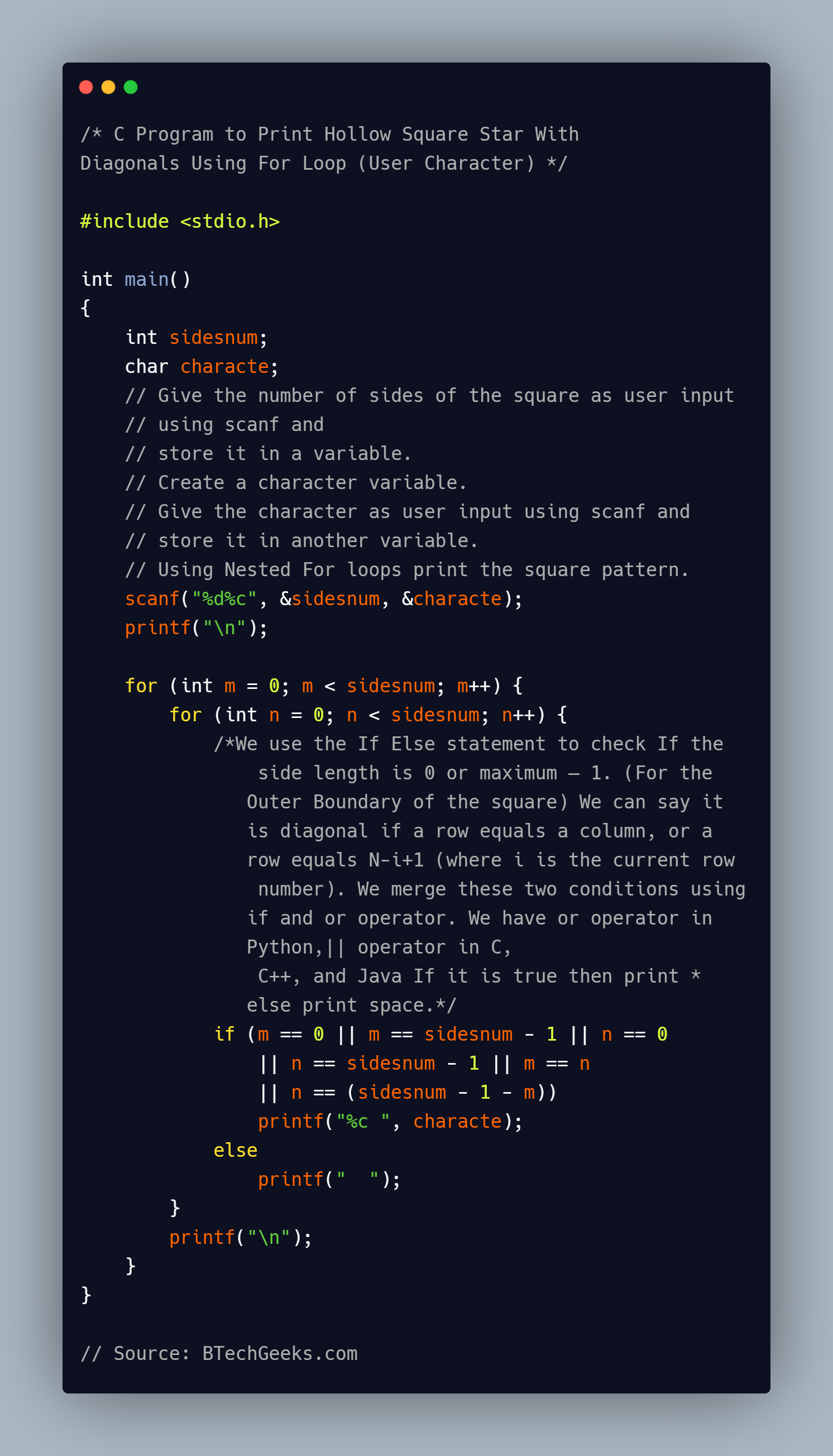#include <stdio.h> int main() { int sidesnum; char characte; // Give the number of sides of the square as user input // using scanf and // store it in a variable. // Create a character variable. // Give the character as user input using scanf and // store it in another variable. // Using Nested For loops print the square pattern. scanf("%d%c", &sidesnum, &characte); printf("\n"); for (int m = 0; m < sidesnum; m++) { for (int n = 0; n < sidesnum; n++) { /*We use the If Else statement to check If the side length is 0 or maximum – 1. (For the Outer Boundary of the square) We can say it is diagonal if a row equals a column, or a row equals N-i+1 (where i is the current row number). We merge these two conditions using if and or operator. We have or operator in Python,|| operator in C, C++, and Java If it is true then print * else print space.*/ if (m == 0 || m == sidesnum - 1 || n == 0 || n == sidesnum - 1 || m == n || n == (sidesnum - 1 - m)) printf("%c ", characte); else printf(" "); } printf("\n"); } }  Output: 10$
    
                   
               
        
   
 
   
          
                
    

Related Programs: